# Test: More On Free Body Problems

## 10 Questions MCQ Test Physics Class 11 | Test: More On Free Body Problems

Description
This mock test of Test: More On Free Body Problems for Class 11 helps you for every Class 11 entrance exam. This contains 10 Multiple Choice Questions for Class 11 Test: More On Free Body Problems (mcq) to study with solutions a complete question bank. The solved questions answers in this Test: More On Free Body Problems quiz give you a good mix of easy questions and tough questions. Class 11 students definitely take this Test: More On Free Body Problems exercise for a better result in the exam. You can find other Test: More On Free Body Problems extra questions, long questions & short questions for Class 11 on EduRev as well by searching above.
QUESTION: 1

### Free body diagram of a situation is shown below. The net force is known, however, the magnitude of few of the forces is not known. The magnitude of the unknown forces C and D will be: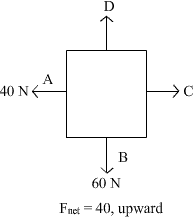Solution: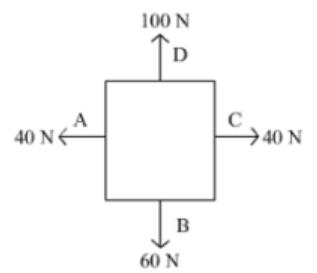Force on A’ and B’ is 40 N and 60 N respectively. Net force of the situation is 40 N upward. So to balance the force on A’ a force of 40 N has to apply on C’. Similarly a force of 100 N is also be applied to get the net force of 40 N in upward direction.

QUESTION: 2

### If a block moving up at an inclined plane with θ =30° with a velocity 5 m/s , stops after 0.5 sec , then coefficient of friction is:

Solution:

The initial velocity is 5 m/s and the block stops after ½ sec,
Hence the net negative acceleration is 10 m/s2
The net force active downwards parallel the plane is mg sin 30° + kmg cos 30°
Hence we get a = g ( sin 30° + kcos 30°) = 10
Thus we get  sin 30° + kcos 30° = 1
k = sec 30° - tan 30°
= 2/1.73 - 1/1.73
= 1/1.73
= 0.6

QUESTION: 3

### A person is sliding an iron box across the floor. Its free body diagram will be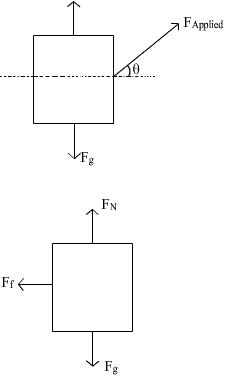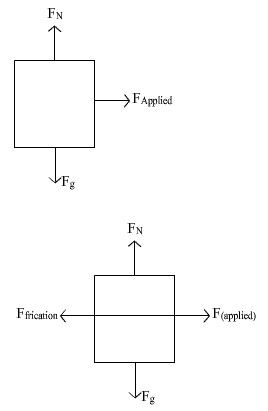Solution:

In the given condition, we get that a component of applied force acts parallel to force rightwards and one is perpendicular. Also the weight, normal and friction (leftwards) would also act upon it
Hence the correct Ans is D.

QUESTION: 4

Free body diagram of a box pushed with constant velocity along a rough horizontal surface is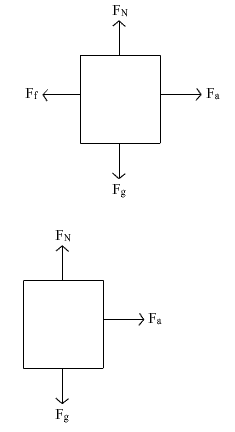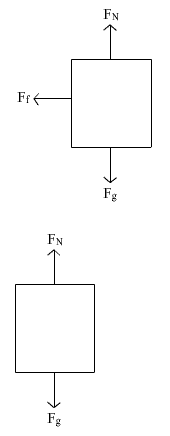Solution:

For a body to move with constant velocity the net force on body should be zero.Only option A can have a net force zero rather b,c net horizontal force is non-zero and in option d there is no horizontal force so no motion in horizontal direction So option a is most appropriate

QUESTION: 5

A lift of mass 1000kg , which is moving with an acceleration of 1 m/s2 in upward direction has tension developed in its string equal to:

Solution:

If we make the fbd of a 1000kg block pulled by a string having tension T,
We get the equation
T - Mg = Ma
Where M = 1000kg and a = 1m/s2
Thus we get, T = M(a + g) = 1000x11 = 11000N

QUESTION: 6

25 N force is required to raise 75 kg mass from a pulley. If rope is pulled 12 m , then the load is lifted to 3 m , the efficiency of pulley system will be:

Solution:

η=total work/useful work= F x d /mgh =250×12/(75g)×3=0.75=75%

QUESTION: 7

An ice cart of mass 60 kg rests on a horizontal snow patch with coefficient of static friction 1/3 . Assuming that there is no vertical acceleration , find the magnitude of maximum horizontal force required to move the ice cart.

Solution: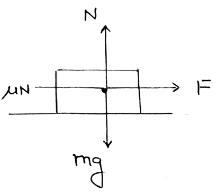Let F be the min. force required to move the cart
then F = μN (along x axis )
Δ N = mg (along y x axis )
⇒ F = μmg = 1/3 x 60 x 9.8
⇒ F = 196 N.

QUESTION: 8

A mass of 100 kg is resting on a rough inclined plane of 60o. If the coefficient of friction is 0.5, then the least force acting parallel to the plane to keep the mass in equilibrium is

Solution:

The force parallel to the plane but downwards, W = mg.sin 60 = 980 x √3/2 = 848.7N
Maximum friction force acting, f = mg.cos 60 x 0.5 = 980 x ½ x 0.5 = 245N
Thus the minimum extra force required, let say F = 848.7 - 245 = 603.3 N

QUESTION: 9

Three blocks connected together lie on a horizontal frictionless table and pulled to the right with a force of F = 100 N. If m1 = 10 kg, m2 = 20 kg, m3 = 30kg. Then the tension T1 and T2 will be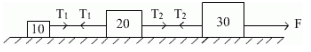Solution:

Considering the three block system, we have
100 = 60.a
where a is the common acceleration of all the blocks
a = 5/3 m/s2
Considering mass 1 we get,
T1 = 10 a
T1 = 50/3 N
Considering mass 1 and 2 we get,
T2  = 30 a
T2 = 50N

QUESTION: 10

Block A has mass 10 kg and block B has mass 5 kg. They are connected by an inextensible rope which passes over the mass less and frictionless pulley. Select the correct free body diagrams for two blocks from the given options.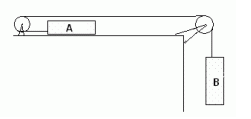Solution:

On the block A, the tension acts leftwards while friction acts rightwards due to normal upwards which balances weight downwards. Similarly the tension acts upwards on block B which balances its weight.